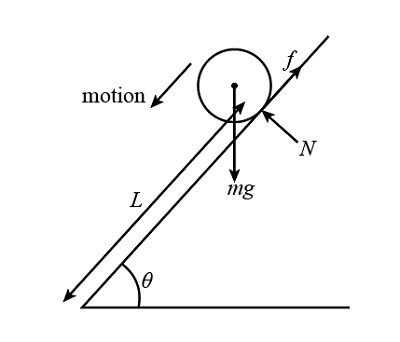# A solid sphere of mass m is placed on a rough incline plane. The coefficient of friction \mu is...

## Question:

A solid sphere of mass m is placed on a rough incline plane. The coefficient of friction {eq}\mu {/eq} is insufficient to start pure rolling. The sphere slides length L from rest and its KE becomes K. Find work done by friction.

## Kinetic energy :

The kinetic energy is found, when the object is in motion. Kinetic energy is part of the energy, which can be transformed according to our needs. When a body falls from a height, it has kinetic energy.

Given data

• The mass of the sphere is: {eq}m {/eq}
• The coefficient of the friction is: {eq}\mu {/eq}
• The length on which sphere slides: {eq}L {/eq}
• The kinetic energy of the system is: {eq}K {/eq}

The free body diagram of the sphere plane system is as follows.The work energy theorem of the system is as follows.

{eq}{W_{friction}} + {W_{gravity}} + {W_{normal\;force}} = K {/eq}

Here, Work done by the normal forces will be zero because of motion direction is the perpendicular to the normal forces.

Work done by the gravity is as follows.

{eq}{W_{gravity}} = mgL\sin \theta {/eq}

Here, g is the gravitational acceleration.

Substitute all the value in above equation.

{eq}\begin{align*} {W_{friction}} + mgL\sin \theta + 0 &= K\\ {W_{friction}} &= K - mgL\sin \theta \end{align*} {/eq}

Thus, the work done by the friction is {eq}{W_{friction}} = K - mgL\sin \theta {/eq}